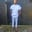Related Tags

laravel
php
communitycreator

# How to get the read time of a string in PHPChinweuba Elijah Azubuike

### Overview

On many platforms like Edpresso, while reading an article, you will see the estimated read time of the article.

In this shot, you will learn how to make a read time in a fast and straightforward way.

Research has found that it takes an adult one minute to read two hundred words, on average. So, we will write our logic based on this fact.

### Methods used

We will use two main methods.

1. str_word_count() is a PHP function that helps count the words.

2. round() method is used to round numbers to their nearest whole number.

### Code

You can paste the following code into any of your controller’s functions or create a function in your controller.

In the following code block, I first counted the number of the words in string $text. Then, I divided it by 200, which is the number of words that can be read in a minute. I did this inside the round() method so we don’t get decimals. I used a conditional statement to check that the time is at least more than a minute. ### Output Please run the below code to get the output: <?php$text = ' Words from your database or words from the request it can be from anywhere';// The text variable string you are working with

$wordCount = str_word_count($text);// getting the number of words
$minutesToRead = round($wordCount / 200);// getting the number of minutes

if($minutesToRead < 1){// if the time is less than a minute$minutes = 'less than a minute';
}else{
$minutes =$minutesToRead;// saving the time in the variable
}
echo \$minutes;
Get the read time of a string

RELATED TAGS

laravel
php
communitycreator

CONTRIBUTORChinweuba Elijah Azubuike
RELATED COURSES

View all Courses

Keep Exploring

Learn in-demand tech skills in half the time